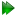SubsectionsSynopsis

Read spin specific parameter values from a file.

Defaults

value.read(param=None, scaling=1.0, file=None, dir=None, spin_id_col=None, mol_name_col=None, res_num_col=None, res_name_col=None, spin_num_col=None, spin_name_col=None, data_col=None, error_col=None, sep=None, spin_id=None)

Keyword arguments

param: The parameter. Only one parameter may be selected.

scaling: The factor to scale parameters by.

file: The name of the file containing the values.

dir: The directory where the file is located.

spin_id_col: The spin ID string column (an alternative to the mol, res, and spin name and number columns).

mol_name_col: The molecule name column (alternative to the spin_id_col).

res_num_col: The residue number column (alternative to the spin_id_col).

res_name_col: The residue name column (alternative to the spin_id_col).

spin_num_col: The spin number column (alternative to the spin_id_col).

spin_name_col: The spin name column (alternative to the spin_id_col).

data_col: The RDC data column.

error_col: The experimental error column.

sep: The column separator (the default is white space).

spin_id: The spin ID string to restrict the loading of data to certain spin subsets.

Description

The spin system can be identified in the file using two different formats. The first is the spin ID string column which can include the molecule name, the residue name and number, and the spin name and number. Alternatively the molecule name, residue number, residue name, spin number and/or spin name columns can be supplied allowing this information to be in separate columns. Note that the numbering of columns starts at one. The spin ID string can be used to restrict the reading to certain spin types, for example only 15N spins when only residue information is in the file.

If this is used to change values of previously minimised parameters, then the minimisation statistics (chi-squared value, iteration count, function count, gradient count, and Hessian count) will be reset.

Relaxation curve fitting parameters

Please see Table 17.27 on page.

Model-free parameters

Please see Table 17.28 on page.

Setting a parameter value may have no effect depending on which model-free model is chosen. For example if S2f values and S2s values are set but the data pipe corresponds to the model-free model `m4' then because these data values are not parameters of the model they will have no effect.

Note that the Rex values are scaled quadratically with field strength and should be supplied as a field strength independent value. Use the following formula to obtain the correct value:

value = rex / (2.0 * pi * frequency) ** 2

where:

 rex is the chemical exchange value for the current frequency. frequency is the proton frequency corresponding to the data.

Reduced spectral density mapping parameters

Please see Table 17.29 on page.

In reduced spectral density mapping, the CSA value must be set prior to the calculation of spectral density values.

Consistency testing parameters

Please see Table 17.30 on page.

In consistency testing, the CSA value, angle Theta (`orientation') and global correlation time must be set prior to the calculation of consistency functions.

Relaxation dispersion parameters

Please see Table 17.32 on page.

Any of the relaxation dispersion parameters which are of the `float' type can be set. Note that setting values for parameters which are not part of the model will have no effect.

Prompt examples

To load 15N CSA values from the file `csa_values' in the directory `data', where spins are only identified by residue name and number, type one of the following:

[numbers=none]